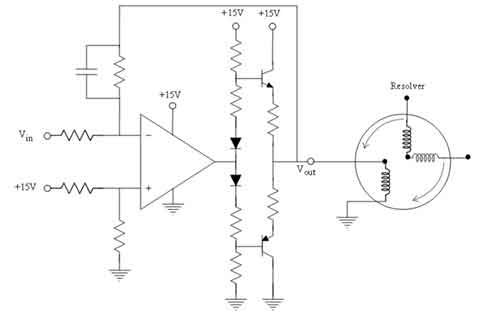#### 逐步搞定波形发生器，手把手如何实现任意波形发生器

##### 日期： 2019-10-28

DDS是从相位的概念直接合成所需波形的一种频率合成技术。不仅可以产生不同频率的正弦波，而且可以控制波形的初始相位。1、顶层模块

2、相位累加器模块

case(choose_wave) 2‘b00:begin

sin_ena <= 1’b1;

cos_ena <= 1‘b0;

sawtooth_ena <= 1’b0;

triangle_ena <= 1‘b0;

end

2’b01:begin

cos_ena <= 1‘b1;

sin_ena <= 1’b0;

sawtooth_ena <= 1‘b0;

triangle_ena <= 1’b0;

end

2‘b10:begin

sin_ena <= 1’b0;

cos_ena <= 1‘b0;

sawtooth_ena <= 1’b1;

triangle_ena <= 1‘b0;

end

2’b11:begin

sin_ena <= 1‘b0;

cos_ena <= 1’b0;

sawtooth_ena <= 1‘b0;

triangle_ena <= 1’b1;

end

default:begin

sin_ena <= 1’b0;

cos_ena <= 1‘b0;

sawtooth_ena <= 1’b0;

triangle_ena <= 1‘b0;

end

endcase

3、查找表

1) 首先使用数学工具计算得到波形采样点，生成mif文件

2) 导入数据采样点，给每一个采样点分配地址，并提供外部寻址的接口，此过程可以通过Quartas Ⅱ来辅助完成

void main(){ int i，j = 0;;

FILE *fp;

fp=fopen(“data.txt”，“w”);

for(i=0;i<=256;i++){

//j=255*sin(2*3.14159/256*i)+0.5;//四舍五入

fprintf(fp，“i=%d，\tj=%d\n”，i，j); }

fclose(fp); }